Kaslo Bull Trout Productivity 2020

The suggested citation for this analytic appendix is:

Thorley, J.L., Amies-Galonski, E. and Dalgarno, S.I.J. (2020) Kaslo Bull Trout Productivity 2020. A Poisson Consulting Analysis Appendix. URL: https://www.poissonconsulting.ca/f/1283025452.

Background

The Kaslo River and Keen Creek, which is a tributary of the Kaslo River, are important Bull Trout spawning and rearing tributaries on Kootenay Lake. From 2012 to 2020, field crews have night-snorkeled these systems in the fall and recorded all juvenile Bull Trout less than 350 mm in length. Keen Creek was not snorkelled in 2020 due to visibility issues. Snorkel and electrofishing marking crews have also captured and tagged juvenile Bull Trout for the snorkel crews to resight. Redd counts have been conducted in both systems since 2006, with the exception of 2020, in which Keen Creek was not surveyed. The primary goal of the current analyses is to answer the following questions:

What is the observer efficiency when night-snorkeling for juvenile Bull Trout in the Kaslo River and Keen Creek?

What are the densities of age-1 Bull Trout in the Kaslo River and Keen Creek?

What is the relationship between the number of redds and the resultant densities of age-1 Bull Trout two years later?

Data Preparation

The data were cleaned, tidied and databased using R version 4.0.3 .

Statistical Analysis

Model parameters were estimated using Maximum-Likelihood (ML) and Bayesian methods. The Maximum-Likelihood Estimates (MLE) were obtained using TMB while the Bayesian estimates were produced using JAGS . For additional information on ML and Bayesian estimation the reader is referred to and , respectively.

Unless indicated otherwise, the Bayesian analyses used uninformative normal prior distributions . The posterior distributions were estimated from 1500 Markov Chain Monte Carlo (MCMC) samples thinned from the second halves of 3 chains . Model convergence was confirmed by ensuring that $$\hat{R} \leq 1.1$$ and $$\textrm{ESS} \geq 150$$ for each of the monitored parameters . Where $$\hat{R}$$ is the potential scale reduction factor and $$\textrm{ESS}$$ is the effective sample size.

The parameters are summarised in terms of the point estimate, lower and upper 95% credible limits (CLs) and the surprisal s-value . The estimate is the median (50th percentile) of the MCMC samples while the 95% CLs are the 2.5th and 97.5th percentiles. The s-value can be considered a test of directionality. More specifically it indicates how surprising (in bits) it would be to discover that the true value of the parameter is in the opposite direction to the estimate. An s-value of 4.3 bits, which is equivalent to a p-value of 0.05, indicates that the surprise would be equivalent to throwing 4.3 heads in a row.

Model averaging and selection was implemented using an information theoretic approach . ML models were compared using the marginal Akaike’s Information Criterion corrected for small sample size (AICc, Burnham and Anderson 2002). The support for fixed terms in models with identical random effects was assessed using the Akaike’s weights ($$w_i$$) . The best supported model was then refitted as its Bayesian equivalent.

Where relevant, model adequacy was confirmed by examination of residual plots for the full model(s).

The results are displayed graphically by plotting the modeled relationships between particular variables and the response(s) with the remaining variables held constant. In general, continuous and discrete fixed variables are held constant at their mean and first level values, respectively, while random variables are held constant at their typical values (expected values of the underlying hyperdistributions) . When informative the influence of particular variables is expressed in terms of the effect size (i.e., percent change in the response variable) with 95% confidence/credible intervals .

The analyses were implemented using R version 4.0.3 and the mbr family of packages.

Model Descriptions

Length Correction

The annual bias (inaccuracy) and error (imprecision) in observer’s fish length estimates when spotlighting (standing) and snorkeling were quantified from the divergence of their length distribution from the length distribution for JLT and SH (the two most experience snorkelers) in that year. More specifically, the length correction that minimised the Jensen-Shannon divergence (Lin 1991) provided a measure of the inaccuracy while the minimum divergence (the Jensen-Shannon divergence was calculated with log to base 2 which means it lies between 0 and 1) provided a measure of the imprecision.

Observer Efficiency

All resighted fish with a tag were allocated to the closest unallocated marked fish (with the same colour tag) by fork length and distance. The marked fish were analysed using a logistic regression model. Key assumptions of the full Maximum Likelihood logistic regression include:

• The observer efficiency varies by the fork length as a second order polynomial.
• The observer efficiency varies by observer.
• The observer efficiency varies between systems.
• The observer efficiency varies by the gradient, sinuosity and river kilometre.

Preliminary analysis indicated that river kilometre received similar support to watershed area.

The final Bayesian logistic regression assumed that:

• The observer efficiency varies by the fork length.

Lineal Density

Both systems were broken into 100 m sites by bank. The lineal density at each site was estimated using an over-dispersed Poisson Generalized Linear Mixed Model. Key assumptions of the full Maximum Likelihood GLMM include:

• The lineal density varies between systems and years.
• The lineal density varies randomly by site.
• The lineal density varies by site sinuosity, gradient and river kilometre.
• The observer efficiency for each system is as estimated by the observer efficiency model.

Preliminary analysis indicated that river kilometre was better supported as a predictor of lineal density than watershed area. Preliminary analysis also indicated that autocorrelation (modeled as an AR1 process) was not significant.

The final Bayesian GLMM dropped sinuosity and gradient and took into account the uncertainty in the observer efficiencies as estimated by the observer efficiency model.

Stock-Recruitment

The stock-recruitment relationship was estimated using a Bayesian Beverton-Holt stock-recruitment curve. Key assumptions of the final BH SR model include:

• The strength of density-dependence varies between systems.

Model Templates

Observer Efficiency

Maximum Likelihood
.#include <TMB.hpp>

template<class Type>
Type objective_function<Type>::operator() () {
DATA_VECTOR(Observed);
DATA_FACTOR(Observer);
DATA_FACTOR(System);
DATA_VECTOR(Tagged);
DATA_VECTOR(Length);
DATA_VECTOR(Sinuosity);
DATA_VECTOR(Rkm);

PARAMETER(bSystem);
PARAMETER(bLength);
PARAMETER(bLength2);
PARAMETER(bSinuosity);
PARAMETER(bRkm);

PARAMETER_VECTOR(bObserver);

vector<Type> eObserved = Observed;

int n = Observed.size();

Type nll = 0.0;

for(int i = 0; i < n; i++){
eObserved(i) = invlogit(bObserver(Observer(i)) + bSystem * System(i) + bLength * Length(i) + bLength2 * pow(Length(i), 2) + bGradient * Gradient(i) + bSinuosity * Sinuosity(i) + bRkm * Rkm(i));
nll -= dbinom(Observed(i), Tagged(i), eObserved(i), true);
return nll;

Block 1. Full model.

Bayesian
.model{
bIntercept ~ dnorm(0, 5^-2)
bLength ~ dnorm(0, 5^-2)

for(i in 1:length(Observed)){
logit(eObserved[i]) <-  bIntercept + bLength * Length[i]
Observed[i] ~ dbern(eObserved[i])
}

Block 2. Final model.

Lineal Density

Maximum Likelihood
.#include <TMB.hpp>

template<class Type>
Type objective_function<Type>::operator() () {
DATA_VECTOR(Count);
DATA_VECTOR(Length);
DATA_VECTOR(Coverage);
DATA_VECTOR(Efficiency);
DATA_VECTOR(Dispersion);

DATA_VECTOR(Sinuosity);
DATA_VECTOR(Rkm);

DATA_FACTOR(System);

DATA_FACTOR(Site);
DATA_INTEGER(nSite);
DATA_FACTOR(Year);

PARAMETER_MATRIX(bSystemYear);
PARAMETER_VECTOR(bSite);

PARAMETER(bSinuosity);
PARAMETER(bRkm);

PARAMETER_VECTOR(bDispersion);
DATA_INTEGER(nDispersion);
PARAMETER(log_sDispersion);
PARAMETER(log_sSite);

Type sSite = exp(log_sSite);
Type sDispersion = exp(log_sDispersion);

vector<Type> eDensity = Count;
vector<Type> eCount = Count;
vector<Type> eNorm = pnorm(bDispersion, Type(0), Type(1));
vector<Type> eDispersion = qgamma(eNorm, pow(sDispersion, -2), pow(sDispersion, 2));

Type nll = 0.0;

for(int i = 0; i < nSite; i++){
nll -= dnorm(bSite(i), Type(0), sSite, true);

for(int i = 0; i < nDispersion; i++){
nll -= dnorm(bDispersion(i), Type(0), Type(1), true);

eDensity(i) = exp(bSystemYear(System(i), Year(i)) + bGradient * Gradient(i) + bSinuosity * Sinuosity(i) +  bRkm * Rkm(i) + bSite(Site(i)));
eCount(i) = eDensity(i) * Length(i) * Coverage(i);

nll -= dpois(Count(i), eCount(i) * Efficiency(i) * eDispersion(i), true);

return nll;

Block 3. The full model.

Bayesian
.model{

bEfficiency ~ dnorm(Efficiency, EfficiencySD^-2) T(0, )

for(i in 1:nSystem) {
for(j in 1:nYear) {
bSystemYear[i,j] ~ dnorm(0, 5^-2)
}
}

log_sSite ~ dnorm(0, 5^-2)
log(sSite) <- log_sSite
for(i in 1:nSite) {
bSite[i] ~ dnorm(0, sSite^-2)
}

bRkm ~ dnorm(0, 5^-2)

log_sDispersion ~ dnorm(0, 5^-2)
log(sDispersion) <- log_sDispersion

for(i in 1:length(Count)) {
log(eDensity[i]) <- bSystemYear[System[i],Year[i]] + bRkm * Rkm[i] + bSite[Site[i]]
eDispersion[i] ~ dgamma(sDispersion^-2, sDispersion^-2)
Count[i] ~ dpois(eDensity[i] * Length[i] * Coverage[i] * bEfficiency * eDispersion[i])
}

Block 4. The final model.

Stock-Recruiment

.model {
log_bAlpha ~ dnorm(5, 5^-2)
log(bAlpha) <- log_bAlpha

for(i in 1:nSystem) {
log_bBeta[i] ~ dnorm(0, 5^-2)
log(bBeta[i]) <- log_bBeta[i]
}

log_sRecruits ~ dnorm(0, 5^-2)
log(sRecruits) <- log_sRecruits

for(i in 1:length(Recruits)){
eRecruits[i] <- bAlpha * Stock[i] / (1 + bBeta[System[i]] * Stock[i])
Recruits[i] ~ dlnorm(log(eRecruits[i]), sRecruits^-2)
}
bCarryingCapacity <- bAlpha / bBeta

Block 5. Final model.

Results

Tables

Coverage

Table 1. Total length of river bank counted (including replicates) by system and year.

System Year Length (km)
Kaslo River 2012 10.57 [km]
Kaslo River 2013 13.43 [km]
Kaslo River 2014 11.45 [km]
Kaslo River 2015 7.32 [km]
Kaslo River 2016 11.59 [km]
Kaslo River 2017 9.79 [km]
Kaslo River 2018 8.44 [km]
Kaslo River 2019 7.19 [km]
Kaslo River 2020 10.42 [km]
Keen Creek 2012 1.44 [km]
Keen Creek 2013 0.95 [km]
Keen Creek 2014 0.67 [km]
Keen Creek 2015 0.72 [km]
Keen Creek 2016 0.85 [km]
Keen Creek 2017 1.68 [km]
Keen Creek 2018 3.37 [km]
Keen Creek 2019 1.48 [km]

Observer Efficiency

Table 2. Parameter descriptions.

Parameter Description
bIntercept The intercept for logit(eObserved)
bLength The effect of Length on bIntercept
bLength2 The effect of Length on the effect of Length on bIntercept
bRkm The effect of Rkm on bIntercept
bSinuosity The effect of Sinuosity on bIntercept
eObserved The expected probability of observing an individual
Length The standardized fork length
Observed The number of individuals observed (0 or 1)
Rkm The standardized Rkm
Sinuosity The standardized site-level sinuosity
Tagged The number of tagged individuals (1)
Maximum Likelihood

Table 3. Model averaged parameter estimates and Akaike weights.

term estimate lower upper svalue
bIntercept -1.0808780 -2.3295953 0.1678392 3.4773626
bLength 0.6677614 0.2681462 1.0673766 9.8869492
bLength2 -0.0425534 -0.2839225 0.1988157 0.4546499
bObserver -2.3082666 -55.9343683 51.3178350 0.1004115
bObserver -0.3257582 -1.5238149 0.8722984 0.7512576
bObserver -0.3072008 -1.4105807 0.7961792 0.7728019
bObserver -2.2142360 -51.7735269 47.3450548 0.1043564
bObserver -0.3711566 -2.0796860 1.3373728 0.5771860
bRkm -0.0126214 -0.2351652 0.2099224 0.1336998
bSinuosity -0.0094249 -0.1826084 0.1637587 0.1280685
bSystem -0.0278483 -0.5354908 0.4797943 0.1291396
Bayesian

Table 4. Final parameter estimates.

term estimate lower upper svalue
bIntercept -1.482489 -1.8471861 -1.130028 10.55171
bLength 0.695636 0.3598602 1.076327 10.55171

Table 5. Observer Efficiency estimates for a 123 mm Bull Trout.

System Efficiency EfficiencySD EfficiencyLower EfficiencyUpper
Kaslo River 0.146685 0.0280345 0.0980146 0.2080916

Table 6. Final model summary.

n K nchains niters nthin ess rhat converged
220 2 3 500 1 730 1.003 TRUE

Lineal Density

Table 7. Parameter descriptions.

Parameter Description
bDispersion The random effect of overdispersion
bRkm The effect of Rkm on bSystemYear
bSinuosity The effect of Sinuosity on bSystemYear
bSite The random effect of Site on bSystemYear
bSystemYear The effect of System and Year on log(eDensity)
Count Number of fish counted
Coverage Proportion of site surveyed
Dispersion Factor for random effect of overdispersion
eCount The expected Count
eDensity The expected lineal density
Efficiency The observer efficiency from the observer efficiency model
Length Length of site (m)
log_sDispersion log(sDispersion)
log_sSite log(sSite)
Rkm Standardized Rkm
sDispersion The SD of bDispersion
Sinuosity Standardized sinuosity
Site The site
sSite The SD of bSite
System The system
Year The year
Maximum Likelihood

Table 8. Model averaged parameter estimates and Akaike weights.

term estimate lower upper svalue
bRkm 0.4821795 0.4803001 0.4840588 Inf
bSinuosity 0.0522280 -0.0014249 0.1058808 4.148099
bSystemYear[1,1] -2.6189795 -2.6319910 -2.6059679 Inf
bSystemYear[2,1] -1.1819174 -1.2211456 -1.1426892 Inf
bSystemYear[1,2] -2.8299663 -2.8359006 -2.8240320 Inf
bSystemYear[2,2] -1.5532761 -1.6287772 -1.4777751 Inf
bSystemYear[1,3] -2.9652543 -2.9733626 -2.9571459 Inf
bSystemYear[2,3] -1.1165560 -1.2021444 -1.0309676 476.602014
bSystemYear[1,4] -2.3892524 -2.4011550 -2.3773498 Inf
bSystemYear[2,4] -0.5613352 -0.6416158 -0.4810546 139.586467
bSystemYear[1,5] -2.8324690 -2.8413748 -2.8235633 Inf
bSystemYear[2,5] -2.6859777 -2.7675664 -2.6043891 Inf
bSystemYear[1,6] -2.1253187 -2.1336582 -2.1169792 Inf
bSystemYear[2,6] -1.3239973 -1.3974273 -1.2505673 906.352094
bSystemYear[1,7] -2.5239050 -2.5336409 -2.5141691 Inf
bSystemYear[2,7] -1.6582903 -1.7308275 -1.5857532 Inf
bSystemYear[1,8] -2.6064297 -2.6154131 -2.5974463 Inf
bSystemYear[2,8] -1.7108347 -1.7730932 -1.6485762 Inf
bSystemYear[1,9] -2.5064879 -2.5150875 -2.4978884 Inf
bSystemYear[2,9] -2.5000000 -2.5000000 -2.5000000 Inf
log_sDispersion -0.6680712 -0.6688614 -0.6672810 Inf
log_sSite -0.8655976 -0.8783731 -0.8528222 Inf
Bayesian

Table 9. Parameter estimates.

term estimate lower upper svalue
bEfficiency 0.1433574 0.0776347 0.1994434 10.5517083
bRkm 0.4854707 0.3825696 0.5918251 10.5517083
bSystemYear[1,1] -2.5895614 -3.0108882 -1.9734800 10.5517083
bSystemYear[2,1] -1.0899258 -1.7006902 -0.3491926 7.7443533
bSystemYear[1,2] -2.8079909 -3.2031556 -2.1574889 10.5517083
bSystemYear[2,2] -1.4344209 -2.2396465 -0.5601481 8.2297802
bSystemYear[1,3] -2.9489954 -3.3772655 -2.3043877 10.5517083
bSystemYear[2,3] -0.9883088 -1.8059546 -0.0885136 5.0598552
bSystemYear[1,4] -2.3473965 -2.7798670 -1.6936240 10.5517083
bSystemYear[2,4] -0.4377502 -1.1189441 0.3850282 1.9114633
bSystemYear[1,5] -2.8119805 -3.2278659 -2.1682952 10.5517083
bSystemYear[2,5] -2.6248922 -3.8121141 -1.5371765 10.5517083
bSystemYear[1,6] -2.1045876 -2.4989584 -1.4775135 10.5517083
bSystemYear[2,6] -1.2136227 -1.8452623 -0.4608058 10.5517083
bSystemYear[1,7] -2.4784520 -2.9054474 -1.8367602 10.5517083
bSystemYear[2,7] -1.5623849 -2.1107687 -0.8570326 10.5517083
bSystemYear[1,8] -2.5725677 -3.0121570 -1.9277275 10.5517083
bSystemYear[2,8] -1.6182459 -2.2834911 -0.8053585 10.5517083
bSystemYear[1,9] -2.4768057 -2.8845415 -1.8388689 10.5517083
bSystemYear[2,9] -0.0126652 -9.8285520 9.2786919 0.0057785
log_sDispersion -0.5879317 -0.7658651 -0.4217460 10.5517083
log_sSite -0.8565095 -1.1981595 -0.6182791 10.5517083

Table 10. Model summary.

n K nchains niters nthin ess rhat converged
1101 22 3 500 20 110 1.008 FALSE

Stock-Recruiment

Table 11. Parameter descriptions.

Parameter Description
bBeta Density-dependence
eRecruits Expected value of Recruits
Recruits Number of age-1 Bull Trout
Stock Number of Bull Trout redds
bAlpha Recruits per stock at low stock density
sRecruits SD of residual variation in Recruits
Bayesian

Table 12. Final parameter estimates.

term estimate lower upper svalue
bAlpha 100.4818607 32.3831554 15569.5777983 10.5517083
bBeta 0.4610103 0.0718305 91.5513575 10.5517083
bBeta 0.2240096 0.0027319 53.6633599 10.5517083
bCarryingCapacity 218.8600050 143.6379897 459.8148416 10.5517083
bCarryingCapacity 441.8676218 229.9165126 10995.6324474 10.5517083
log_bAlpha 4.6099745 3.4776377 9.6530696 10.5517083
log_bBeta -0.7743362 -2.6336163 4.5168063 0.9611212
log_bBeta -1.4960666 -5.9030147 3.9825656 1.5830415
log_sRecruits -0.8356215 -1.1613737 -0.3544944 8.2297802
sRecruits 0.4336049 0.3130558 0.7015290 10.5517083

Table 13. Final model summary.

n K nchains niters nthin ess rhat converged
17 10 3 500 100 162 1.022 TRUE

Figures

Fish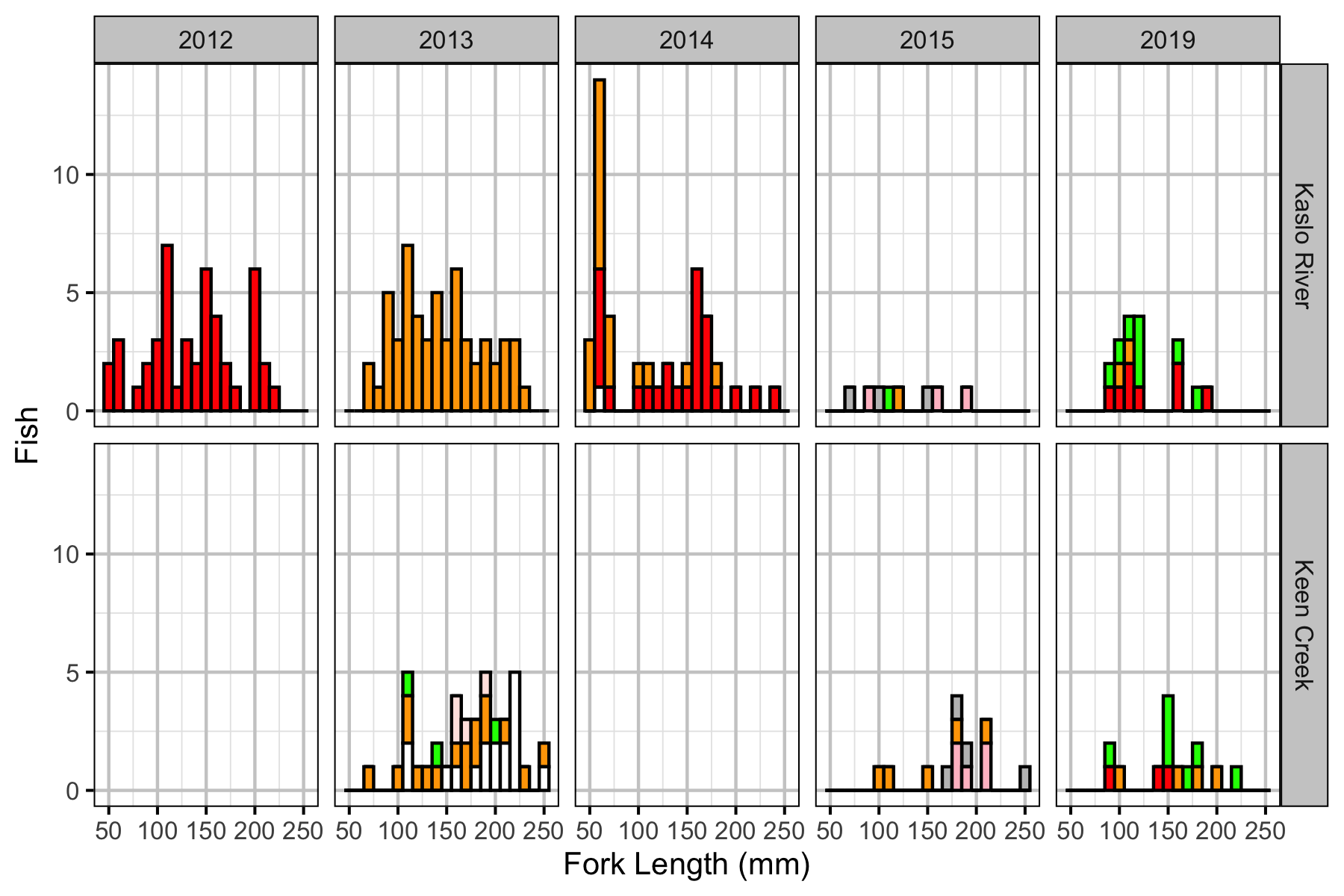Figure 8. Length-frequency plot of marked Bull Trout by year and system.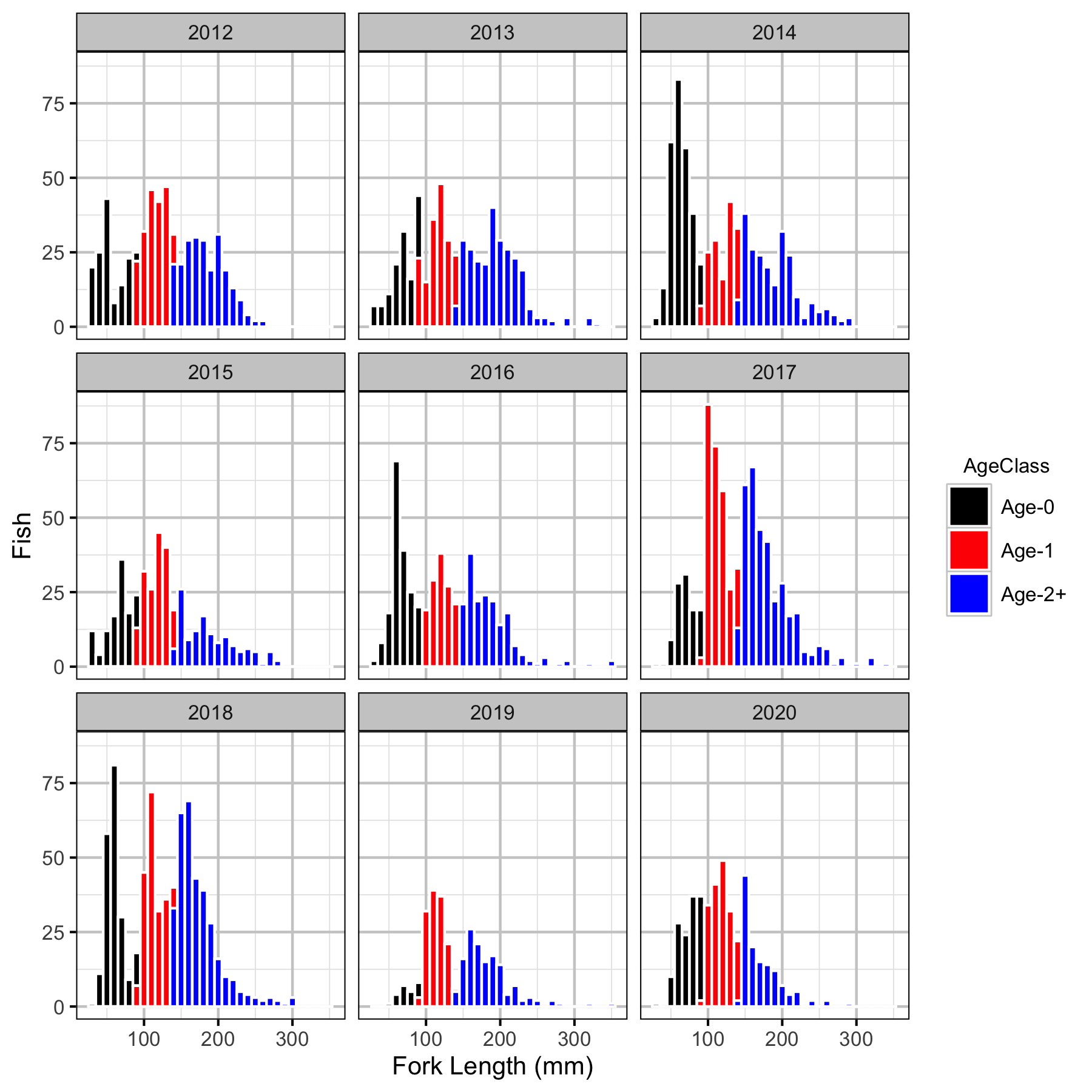Figure 9. Length-frequency plot of observed Bull Trout by year.

Observer Efficiency

Bayesian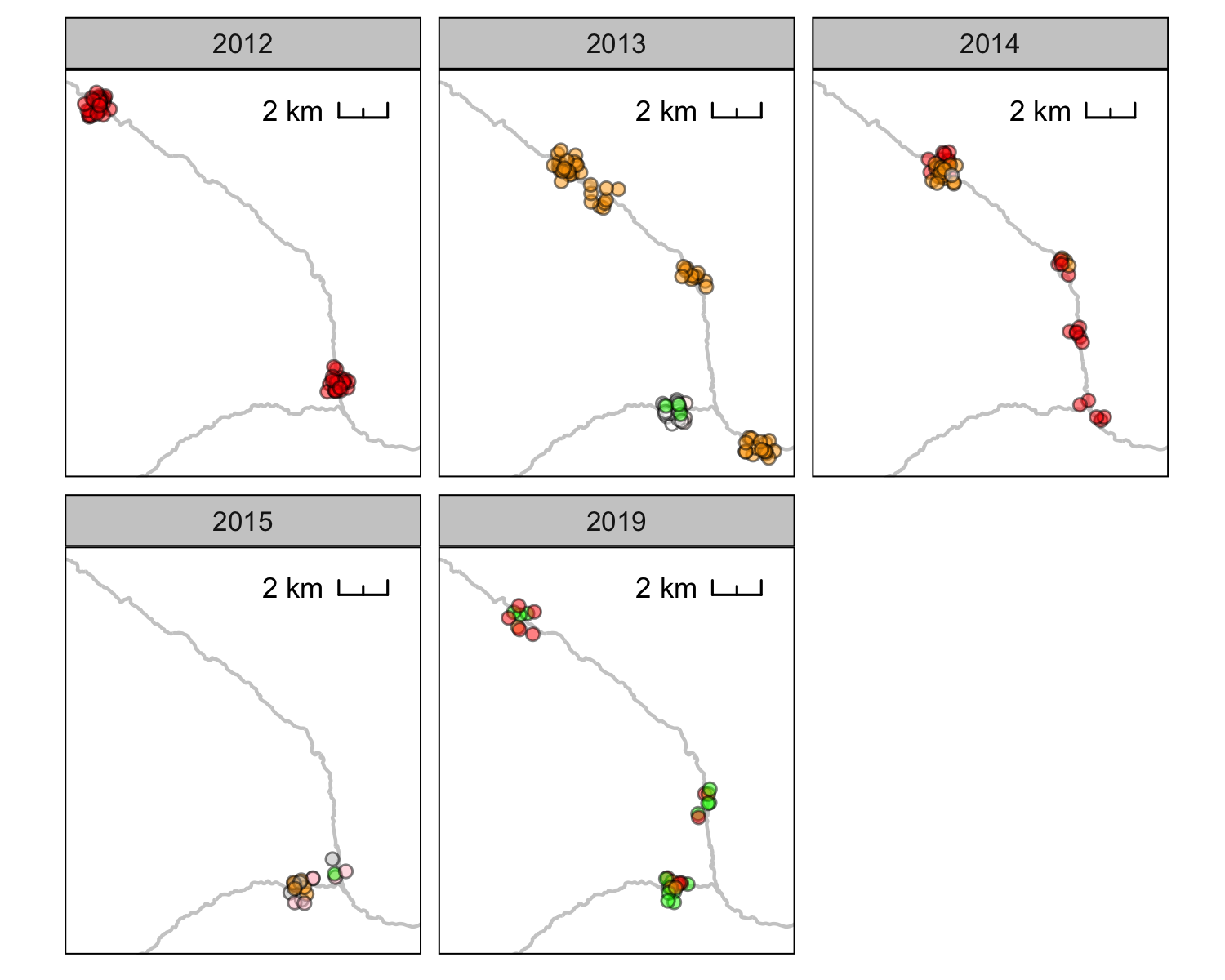Figure 10. Distribution of marked juvenile Bull Trout by year and tag color.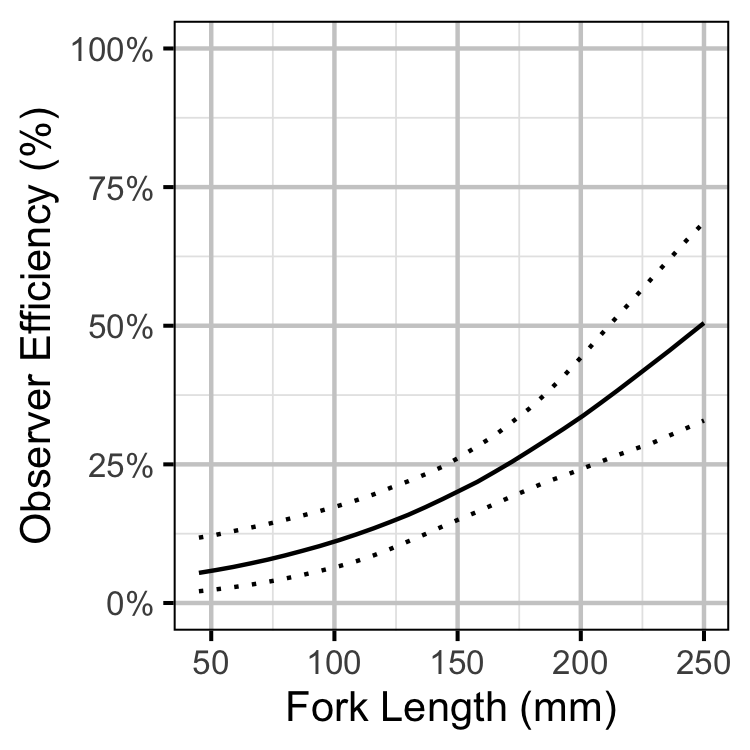Figure 11. Estimated observer efficiency by fork length and system (with 95% CIs).

Lineal Density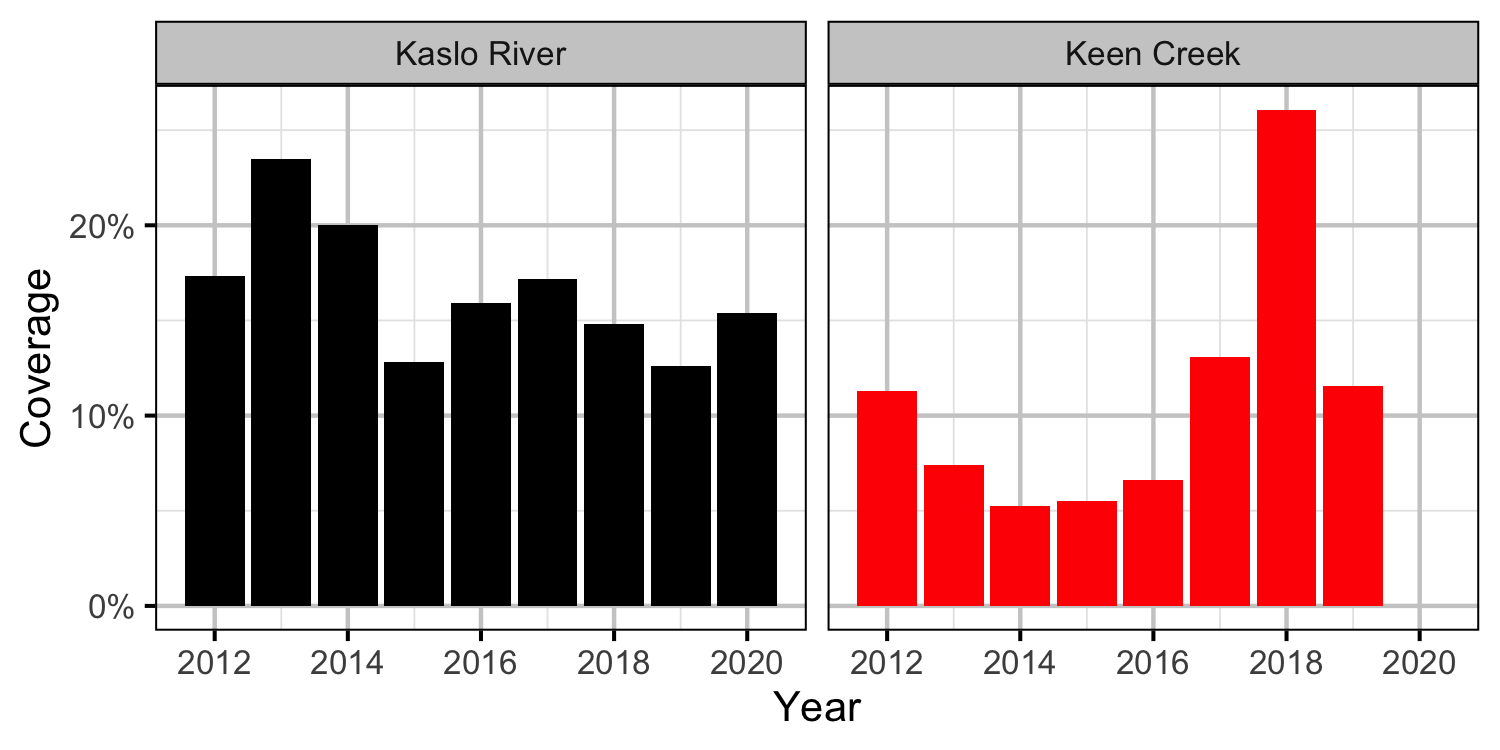Figure 12. Percent coverage by year and system.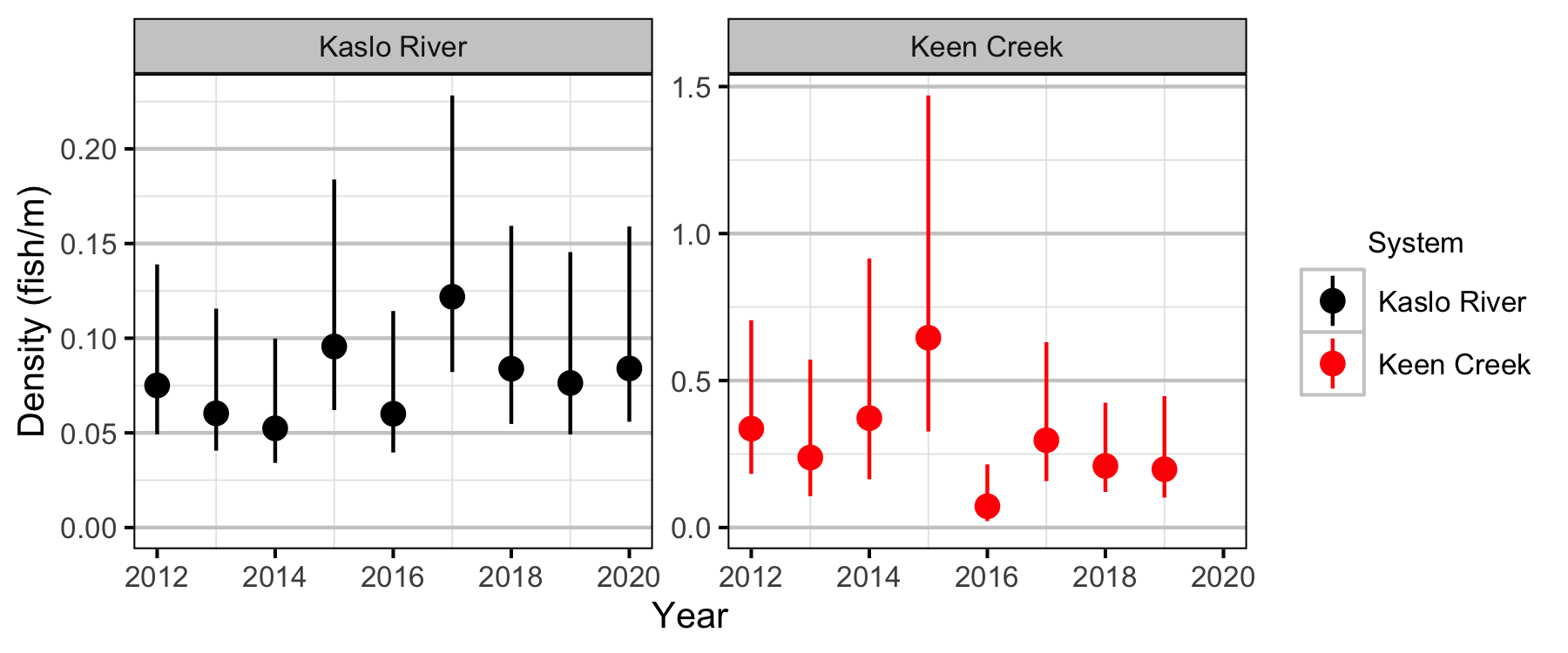Figure 13. Estimated density by year and system (with 95% CIs).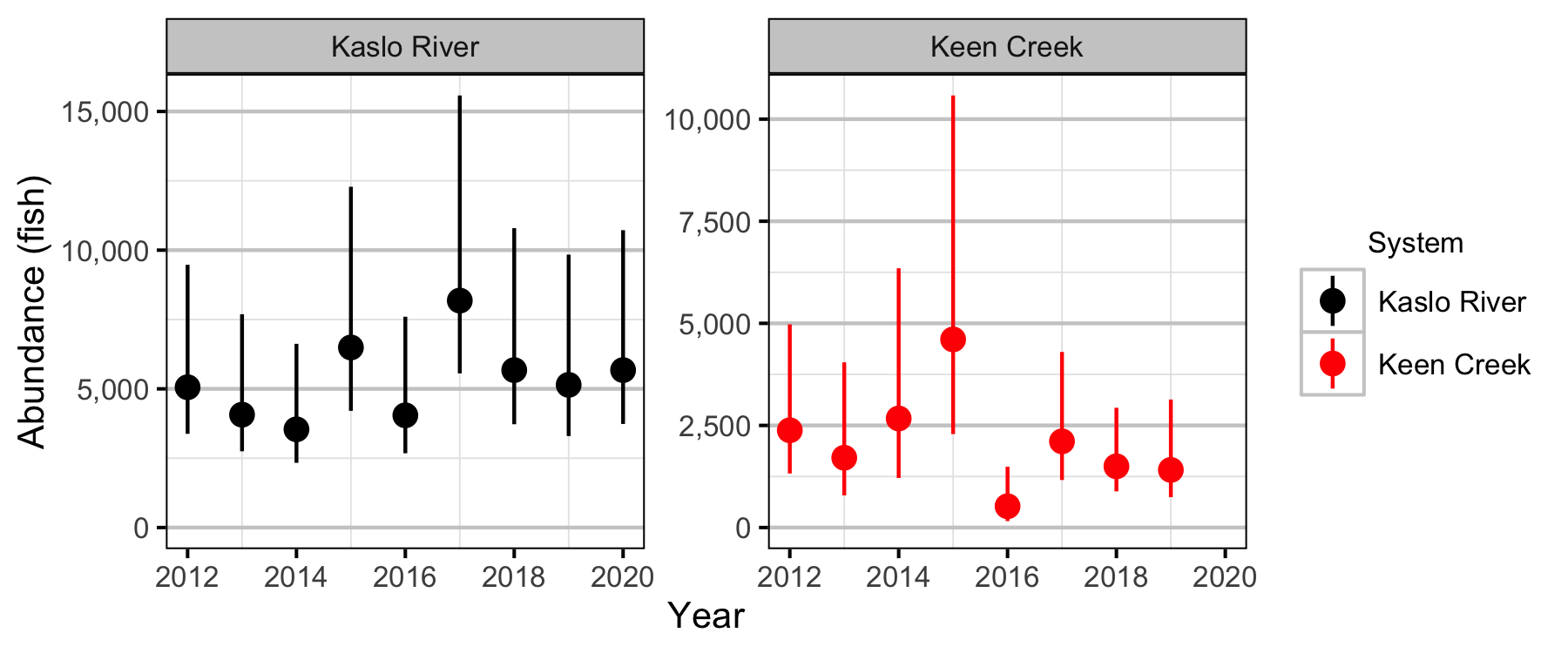Figure 14. The estimated abundance by year and system (with 95% CIs).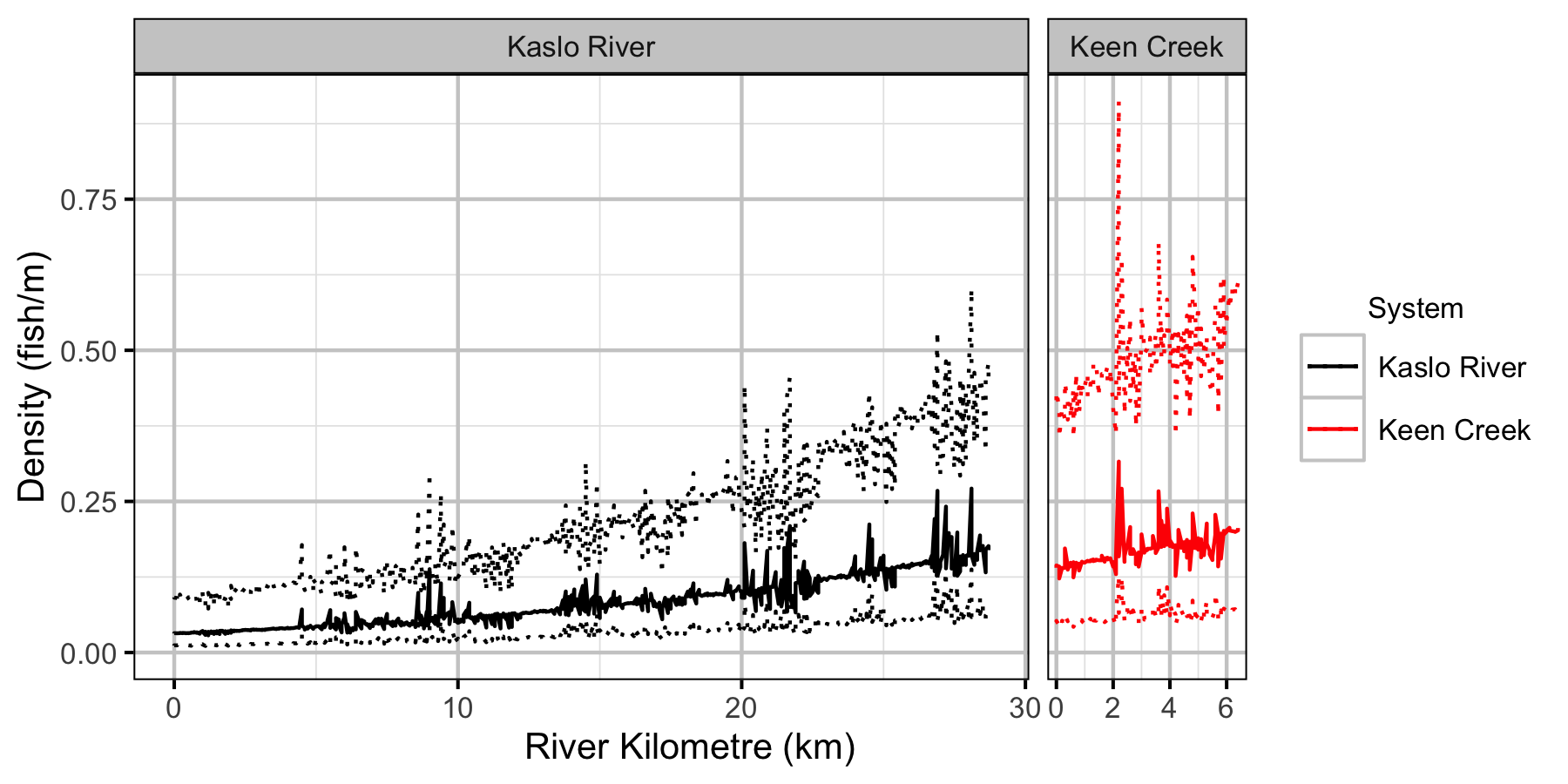Figure 15. The estimated density by site and system (with 95% CIs).

Stock-Recruiment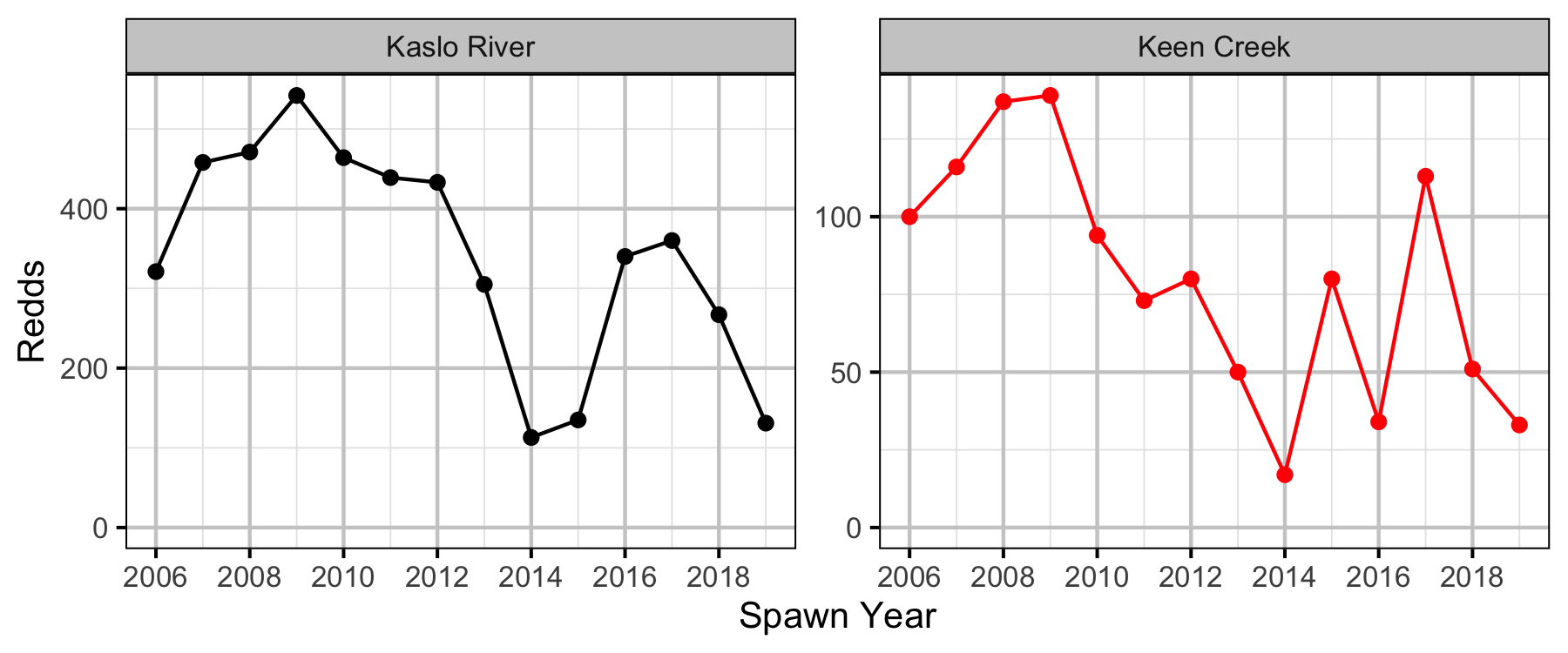Figure 16. Complete redds by system and spawn year.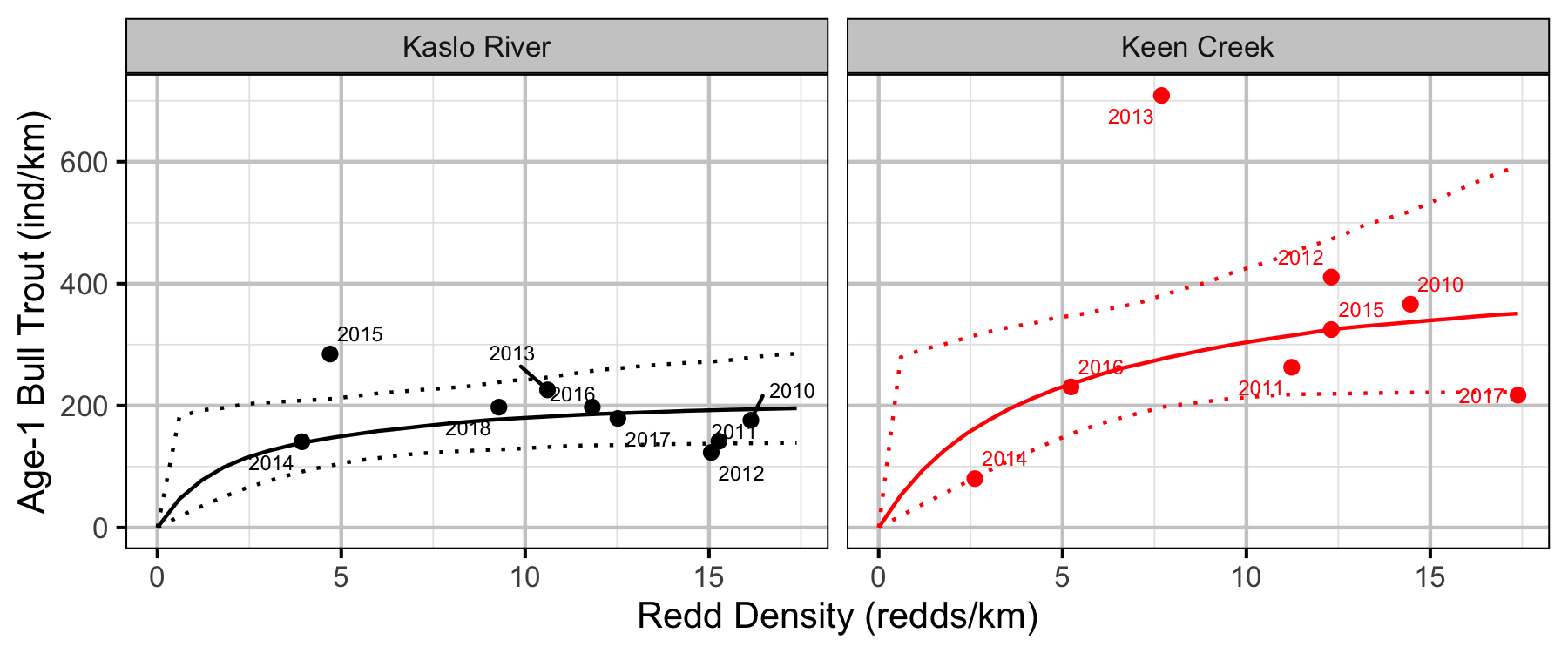Figure 17. Estimated stock-recruitment relationship (with 95% CIs). The points are labelled by spawn year.

Conclusions

• Observer efficiency is approximately 17% for age-1 Bull Trout.
• Age-1 Bull Trout are relatively evenly distributed with respect to mesohabitat.
• Lineal densities of age-1 Bull Trout increase with river kilometre in both systems.
• The age-1 carrying capacity is estimated to be around 220 fish per km in Kaslo River and around 440 fish per km in Keen Creek.

Acknowledgements

The organisations and individuals whose contributions have made this analysis report possible include:

• Habitat Conservation Trust Foundation
• Ministry of Forest, Lands and Natural Resource Operations
• Greg Andrusak
• Ministry of Environment
• Jen Sarchuk
• BC Fish and Wildlife